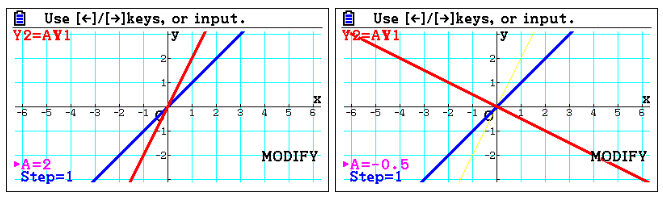Exploring functions with graphing technology on the fx-CG50 - Casio Calculators

# Exploring functions with graphing technology on the fx-CG50

Dec 2022 Medium Read: 4 Min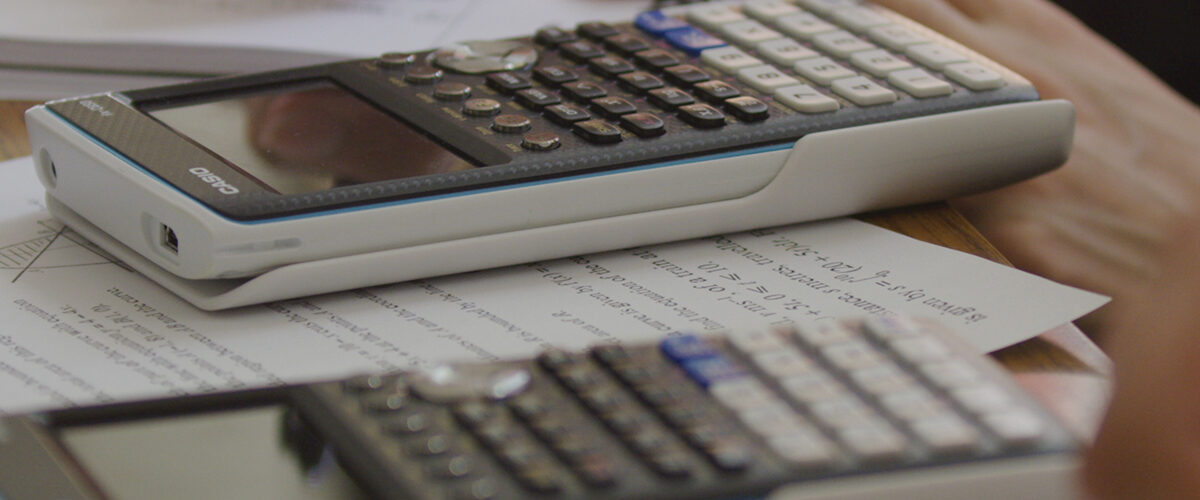Students typically start exploring functions early on in school, often either at primary school or in the first few years at secondary school. However, when starting A Level studies, functions become more challenging, and students begin exploring more complicated aspects of functions. We spoke with mathematician Simon May to explore how graphing technology helps students improve their understanding of functions.

## Starting with a solid foundation

Students are usually familiar with linear functions, for instance, y=2x+1. Inputting this function into the graphing calculator shows them how it plots to a graph and reinforces the discussion around the mappings that functions have: in this case, the one-to-one mapping, where one value of x gives one value of y.

This exploration is taken further by introducing different  functions, like y=x2. Straight away, students will see the visual differences between a quadratic and linear function, where the quadratic has a many-to-one mapping: more than one value of x will give you the same  value of y. Getting students to identify where a vertical  line through the graphed function crosses the x-axis reinforces this. With the visual, students naturally comprehend this relationship more quickly than they would without this aid.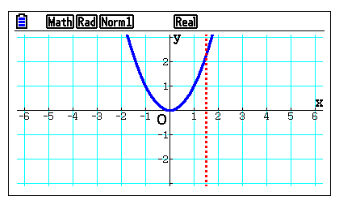Getting students to  explore what type of function y=x could be a useful next step, as they may understand it as undoing a square operation.

Students may be surprised to see that what looks like only part of the graph has been sketched. This is because the calculator will only sketch the expression entered. Students will see that a  vertical line crosses the sketched function once, meaning that this mapping is one-to-one.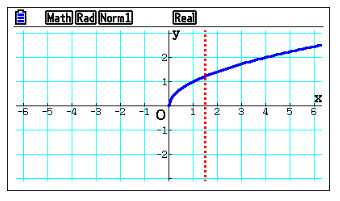## A discussion on semantics

Building on the exploration around y=x naturally introduces the discussion points on semantics and how to treat it as a function. It is a useful reminder to students to be careful in their thinking. In this example, the implication is that what is entered is y=+x, which is a positive number. But, when inputting y=x, they will see that it can be both a positive and a negative number, so one value of x provides more than one value of y, a one-to-many mapping, which the graphical representation makes much clearer. This nicely introduces the concept of a mapping not being a function.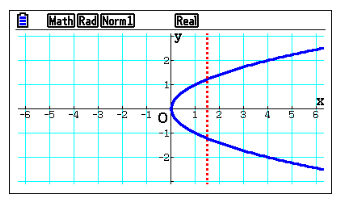## Investigating how changes to functions impact the graph line

A key reason to explore functions with graphing technology is that it’s quick and easy for students to input new scenarios and see the impact these changes have on the line. For example, say they start with y=x; they’ll see this represented on the graph. But what happens when they change this to  y=x + 1? Or y=x +2 ? What is happening to the output of the function?

Using the graphing calculator, they can easily update the function and see how this change is represented on the screen. To take it even further, ask students to predict the changes before they modify them.

A key advantage of using the fx-CG50 is that students can input the starting function and make changes to this function without changing the original. This feature enables faster explorations, as they don’t need to keep inputting the same function multiple times.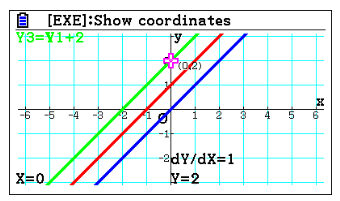## Using modify in graph mode ro explore transforming graphs

The calculator allows students to perform simple transformations, such as multiplying a previously stored function. When doing this action, the function will update on the screen. But it will also leave a faint line where the original function was, which helps students see the impact of the transformation on the line.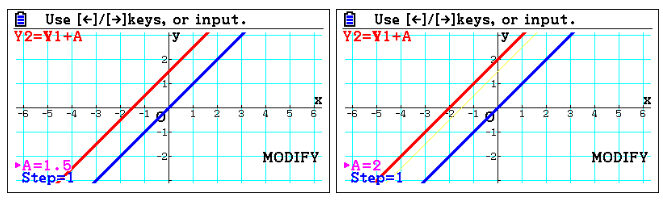One area that students often struggle with is transformations of functions such as the translation 𝑓(x-a). Starting the exploration with a discussion, asking students to predict what will happen and then getting them to perform the function will highlight whether their prediction was right. Explaining it algebraically and then demonstrating it graphically reinforces what is happening and helps students understand how the subtraction of the constant impacts the function.

Students can also perform stretches on functions, for instance, f(ax) . Students can change the value of A and see how this impacts the scale factor. Trying both smaller values, like 0.5, negatives and larger numbers emphasises how the scale factor can increase and decrease and the effect on the sketch.